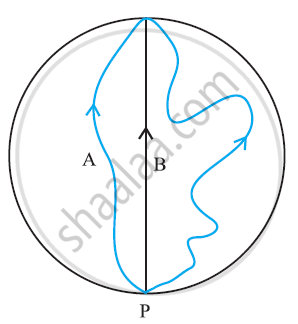CBSE (Science) Class 11CBSE
Share

# Three Girls Skating on a Circular Ice Ground of Radius 200 M Start from a Point Pon the Edge of the Ground and Reach a Point Qdiametrically Opposite to P Following Different Paths What is the Magnitude of the Displacement Vector for Each? for Which Girl is this Equal to the Actual Length of the Path Skated? - CBSE (Science) Class 11 - Physics

#### Question

Three girls skating on a circular ice ground of radius 200 m start from a point Pon the edge of the ground and reach a point Qdiametrically opposite to P following different paths as shown in Figure. What is the magnitude of the displacement vector for each? For which girl is this equal to the actual length of the path skated?#### Solution 1

Displacement is given by the minimum distance between the initial and final positions of a particle. In the given case, all the girls start from point P and reach point Q. The magnitudes of their displacements will be equal to the diameter of the ground.

Radius of the ground = 200 m

Diameter of the ground = 2 × 200 = 400 m

Hence, the magnitude of the displacement for each girl is 400 m. This is equal to the actual length of the path skated by girl B.

#### Solution 2

Displacement for each girl = vec(PQ)

∴ Magnitude of the displacement for each girl

=PQ = diameter of circular ice ground

= 2 x 200 = 400 m.

Is there an error in this question or solution?

#### Video TutorialsVIEW ALL 

Solution Three Girls Skating on a Circular Ice Ground of Radius 200 M Start from a Point Pon the Edge of the Ground and Reach a Point Qdiametrically Opposite to P Following Different Paths What is the Magnitude of the Displacement Vector for Each? for Which Girl is this Equal to the Actual Length of the Path Skated? Concept: Scalars and Vectors.
S